18. Quantitative Reasoning: Question Types & Strategies

Quantitative Reasoning: A High School Reunion

The questions on this section of the GRE cover the algebra, geometry, and data analysis that is taught in high school. If you were not a math major, you can review those skills by watching our video tutorials and/or working with Brainfuse tutors. If you were a math major, you should still get familiar with the 4 types of questions that you will see on the exam:

• Multiple Choice: 1 or More Answers
• Quantitative Comparisons
• Numeric Entries

A Closer Look at the 4 Types of QR Questions

These are traditional multiple-choice questions. If you come up with an answer that is not listed, double check the answer choices to see if it is written in a different form—as a fraction instead of a decimal, for example.

Not there? Try the problem again, or use some of the tips below to solve it:

Example: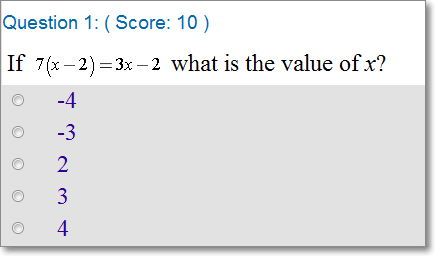How can you solve this problem?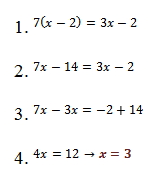II. Multiple Choice: 1 or More Answers

These problems may have more than one correct answer:

• If a multiple-choice question does not tell you exactly how many answer choices to select, there may be more than one right answer.
• If it does tell you how many choices to select, do not disobey this test! It will not respond well. Make sure to choose the exact number it has asked for instead.
• All correct answers need to be selected because the GRE does not give partial credit.
• There still may only be 1 right answer.
• All 5 choices could also be correct.

Example: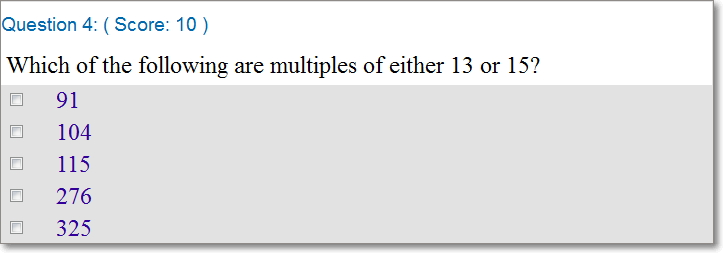How can you solve this problem?

First, we can divide each answer choice by 13. (This should not bring bad luck unless none of the answer choices are divisible by 13.)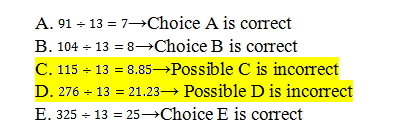Since the problem said “multiples of either 13 or 15,” we can divide the 2 ostracizedchoices by 15.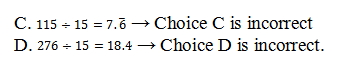Choices A, B, and E are the answers.

III. Quantitative Comparisons

• How does one value compare to another?
• Is that always the case?

These are the two questions that you can ask yourself when faced with a quantitative comparison question.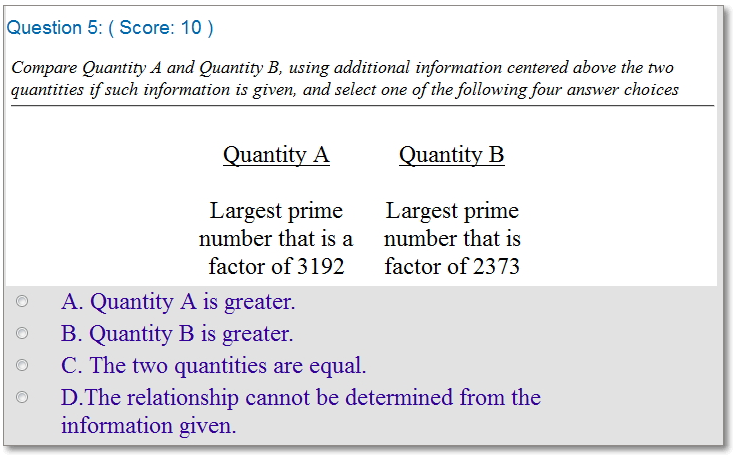These questions always have the same answer choices, and they are always in the same order. So, be sure to memorize them in order to save time on the test.

How can you solve this problem?

Factor both quantities.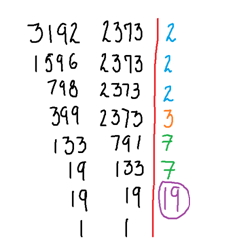Since 19 is a prime number and is the greatest factor for both numbers, the correct answer is C.

Tips:

• Comparing the values may just involve rewriting an expression in a different way
• If there are variables, you can plug simple integers into them, like 1, 0, and -2.
• Some problems may have conditions, like "if x is not equal to 0."  These conditions are often very helpful in helping you compare the two values.

IV. Numeric Entries

There are no answer choices for these questions because you’ll type your solution into a box (or 2 boxes if you have a fraction).

Tips:

2. Plan how to solve the problem.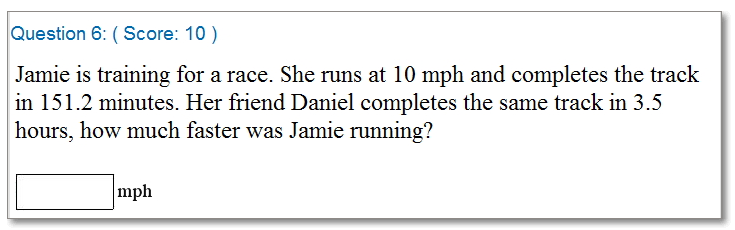How can you solve this problem?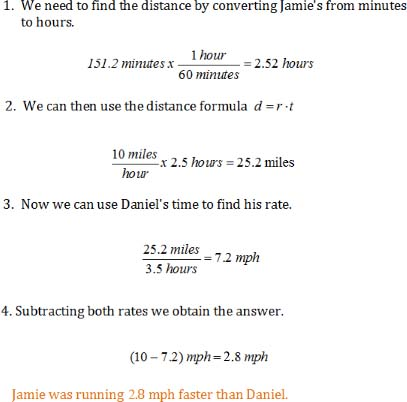These boxes are a feature on the official GRE exam, but they will not be on the Brainfuse tests. To prepare for these questions, please log on to Live Help and let a Brainfuse tutor know that you would like to work on solving numeric entry questions on the GRE.

 GREVocab in action